# High School Math : Isosceles Triangles

## Example Questions

1 2 4 Next →

### Example Question #2 : How To Find The Length Of The Side Of A 45/45/90 Right Isosceles Triangle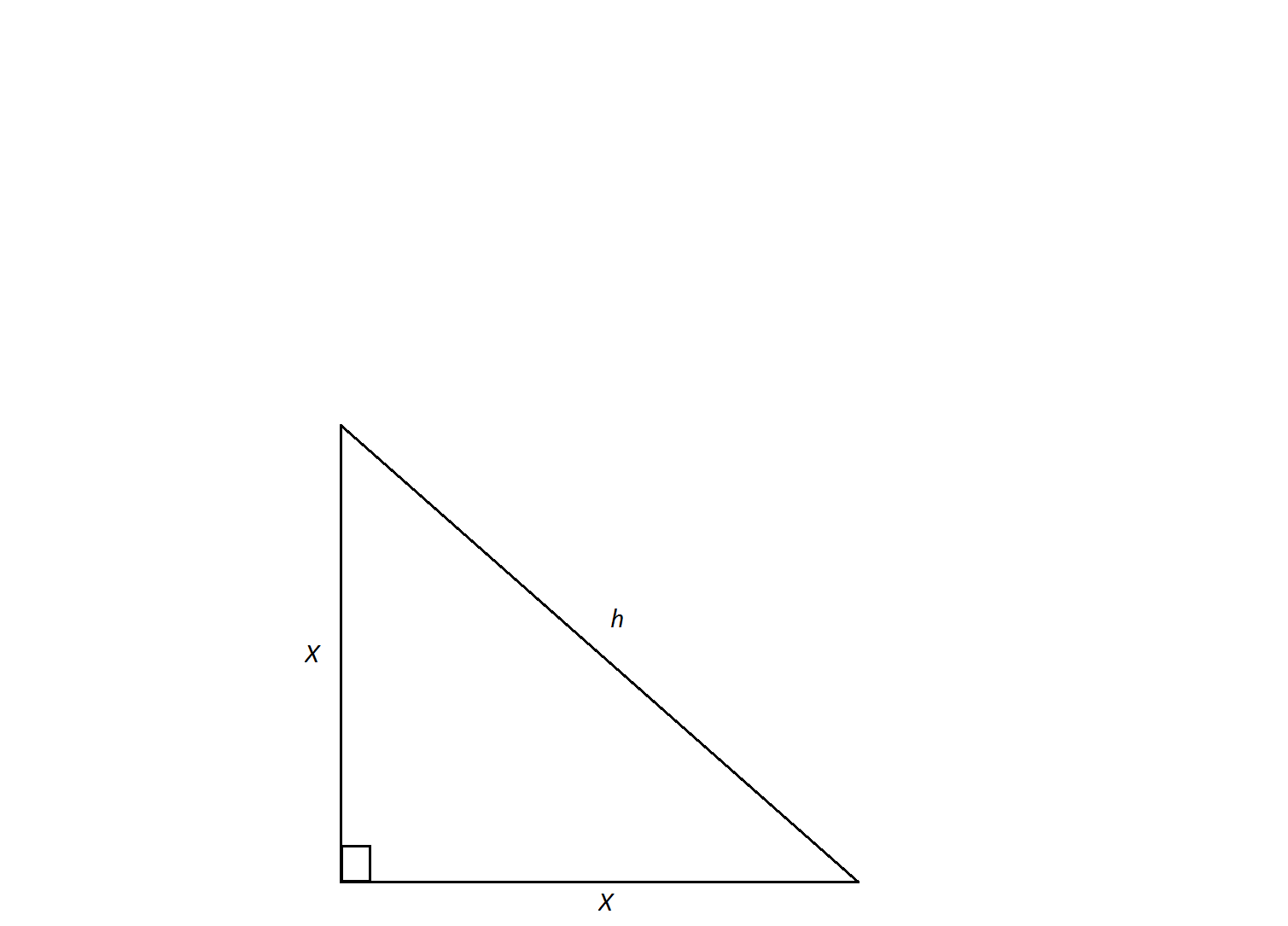The measure of the sides of this isosceles right triangle are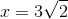. Find the measure of its hypotenuse,.

Possible Answers:Not enough information to solveCorrect answer:Explanation:

An isosceles triangle is a special triangle due to the values of its angles.  These triangles are referred to as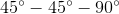triangles and their side lenghts follow a specific pattern that states you can calculate the length of the hypotenuse of an isoceles triangle by multiplying the length of one of the legs by the square root of 2.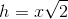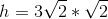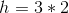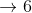1 2 4 Next →

### All High School Math Resources